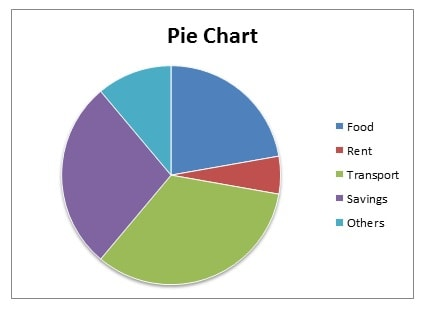# JS2 Mathematics 3rd Term

Word Problems on Algebraic Fractions

David and Doyin share #380.00 in such a way that 1/5 of David’s share is equal to 4/5 of Doyin’s. Find the shares. (Source: MM P114 Ex F Q9)

Solution

Let David’s share = V

Let Doyin’s share = Y

i.e. from the question, David’s share plus Doyin’s share = #380.00

i.e. V + Y = #380.00 — 1

Make Y the subject of the formula in equ (1)

i.e. Y = #380.00 – V — 2

1/5 of David’s share = 1/5 x V        — 3

4/5 of Doyin’s share = 4/5 x Y        — 4

In the question, the fraction of David’s share is equal to the fraction of Doyin’s share.

i.e. 1/5 x V = 4/5 x Y — 5

Cross multiply (CRM)

i.e. 1 x V x 5 = 5 x 4 x Y

i.e. 5V = 20Y — 6

Divide both sides (DBS) by 5

i.e. 5V/5 = 20Y/5

i.e. V = 4Y      — 7

Substitute the value of V in equ (7) into equ (2)

i.e. Y = #380.00 – 4Y           — 8

Collect like terms (CLT)

i.e. Y + 4Y = #380.00

i.e. 5Y = #380.00

Divide both sides (DBS) by 5

i.e. 5Y/5 = #380.00/5

Therefore, Y = #76.00

Substitute the value of Y into equ (7)

i.e. V = 4 x #76.00

Therefore, V = #304.00

Note that you can also substitute the value of Y into equ (2) to solve for the value of V.

Work in Progress

Work in Progress

Work in Progress

Work in Progress

Work in Progress

Work in Progress

Work in Progress

Data Presentation: Frequency Table

 The heights of students in a class in centimetres is given below. 110 112 114 115 116 120 120 120 115 116 111 112 117 118 119 120 119 117 114 113 113 110 111 112 119 110 111 118 119 120 (a) Prepare a frequency table for this distribution (b) Which height occurs most frequently? (c) How many students are measured? MM Pg 193 Q2
 (a) Solution Height(cm) Freq(f) 110 3 111 3 112 3 113 2 114 2 115 2 116 2 117 2 118 2 119 4 120 5 Total Freq 30
 (b) Solution Height 120 cm
 (c) Solution 30 students

Data Presentation: The Pie Chart

 The expenditure pattern of Mr. John working in an oil company per month is as follows. MM Page 196 Q6 Food #40,000.00 Rent #10,000.00 Transport #60,000.00 Savings #50,000.00 Others #20,000.00
 Solution Item Expenditure(N:K) Angle(⁰) Food 40,000.00 80 Rent 10,000.00 20 Transport 60,000.00 120 Savings 50,000.00 100 Others 20,000.00 40 Total 180,000.00 360Work in Progress

 MM P206 Ex B3 Q20 A box contains 40 oranges, 12 of which are unripe. An orange is picked at random. What is the probability that it is: (a) ripe? (b) unripe? Total number of possible outcome = 40 Unripe oranges = 12 Ripe oranges = 40 – 12 (a) number of expected oucome (ripe) = 28 ∴ Prob (of ripe) = 28/40 = 7/10 (b) number of expected oucome (unripe) = 12 ∴ Prob (of unripe) = 12/40 = 3/10

Work in Progress

Work in Progress

Work in Progress

Work in Progress

Work in Progress

Work in Progress

Work in Progress

Work in Progress

Work in Progress

Work in Progress

Work in Progress

Work in Progress

Work in Progress

Work in Progress

Work in Progress

Work in Progress

Work in Progress

Work in Progress

Work in Progress

Work in Progress

# Books & References

error: Content is protected !!